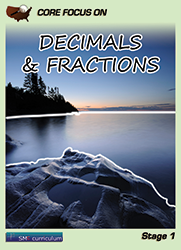#### Decimals & Fractions

Students apply and extend previous understandings of multiplication and division to divide fractions by fractions in this course. They also learn to compute fluently with multi-digit numbers including decimals and find common factors and multiples.

At the end of the course, students solve real-world and mathematical problems involving area, surface area, and volume.

### Textbook & Stage I Series Pricing

1 FREE Teacher Edition(TE) and Teacher Resource Binder(TR) per 40 student texts ordered!